# Boolean functions, normal forms of

Formulas of a special kind realizing Boolean functions. One distinguishes between disjunctive normal forms (cf. Boolean functions, minimization of) and conjunctive normal forms. A product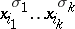, where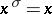if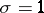andif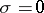, is called an elementary conjunction of rankif all its variables are different; "1" is considered to be an elementary conjunction of rank zero. A logical sum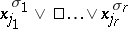is called an elementary disjunction of rankif all its variables are different; "0" is considered to be an elementary disjunction of rank zero.

A formula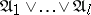, whereare distinct elementary conjunctions of ranks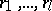, respectively, is called a disjunctive normal form, and the number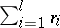is called its complexity; a formula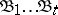, where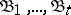are different elementary disjunctions of ranks, respectively, is called a conjunctive normal form, and the numberis called its complexity. Every Boolean function that does not vanish identically can be defined by a disjunctive normal form which is, generally speaking, not unique. The same applies to conjunctive normal forms and functions that do not identically vanish.

From a table defining a Boolean function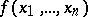one easily obtains the perfect disjunctive normal form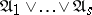, where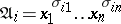,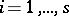, and the sets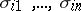are such that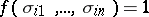. The perfect disjunctive normal form realizing a Boolean functionis unique. The perfect conjunctive normal form is defined similarly.

Since for "almost-all" Boolean functions the number of unit sets varies between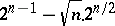and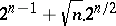, the asymptotic complexity of the perfect disjunctive normal form for "almost-all" Boolean functions is. The maximal complexity of the perfect disjunctive normal forms for functions invariables is attained for functions that vanish at a single point. This complexity is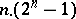.

The main problem in the theory of normal forms of Boolean functions is that of minimizing Boolean functions, i.e. the construction of conjunctive or disjunctive normal forms of minimal complexity — minimal conjunctive or disjunctive normal forms for any given Boolean function (cf. Boolean functions, minimization of; Algorithm, local). Here, by virtue of the duality principle, it is sufficient to consider disjunctive normal forms only. In connection with the problem of minimizing Boolean functions one also considers abridged dead-end, shortest and minimal disjunctive normal forms. An important problem in the theory of disjunctive normal forms is the search for numerical characteristics of them, and characteristics connecting various types of disjunctive normal forms of a given function.

The abridged disjunctive normal form is uniquely constructed from a Boolean function by means of a fairly simple algorithm. Its most important property is the fact that every minimal disjunctive normal form of a function and at least one shortest form can be obtained from the abridged disjunctive normal form by the elimination of certain elementary conjunctions. Therefore many minimization algorithms employ the abridged disjunctive normal form as the initial specification of a Boolean function. It is of major interest in this context to define the complexity of abridged disjunctive normal forms for "almost-all" functions and to determine the absolute maximum of this complexity. Ifis the number of elementary conjunctions in the abridged disjunctive normal form of a Boolean functionand ifthen the following estimates hold:and for "almost-all" Boolean functions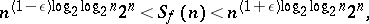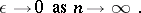It is clear from these results and from the estimates of the complexity of the perfect disjunctive normal form that the complexity of the abridged disjunctive normal form is much higher than that of the perfect disjunctive normal form, both in the "typical" and in the "record-breaking" cases. Unlike the perfect and the abridged disjunctive normal form, a given Boolean function may have many dead-end and minimal disjunctive normal forms. Let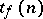be the number of dead-end disjunctive normal forms and let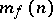be the number of minimal disjunctive normal forms of a Boolean function,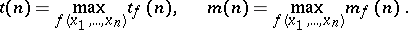Then the following estimates hold: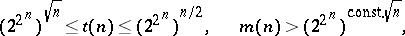and for "almost-all" Boolean functions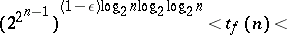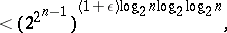A non-trivial upper estimate forand a non-trivial estimate forfor "almost-all" functions are as yet (1977) not known. Complexity estimates of dead-end and of minimal disjunctive normal forms are of great interest in problems of minimization of Boolean functions. Let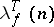be the number of elementary conjunctions in a dead-end disjunctive normal formof the function; let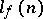be the number of elementary conjunctions in a shortest disjunctive normal form of,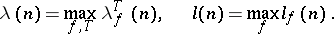The following estimates then hold: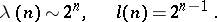For "almost-all" Boolean functionsand for almost-all dead-end disjunctive normal forms: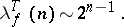For "almost-all" Boolean functions: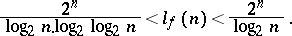These estimates show that the shortest (and also the minimal) disjunctive normal forms of "almost-all" Boolean functions account for only a small fraction of the number of dead-end disjunctive normal forms. There are also estimates of the relative complexity of dead-end and shortest disjunctive normal forms of Boolean functions. Let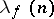be the maximal number of elementary conjunctions in a dead-end disjunctive normal form of a function. For "almost-all" Boolean functionsThe maximum value of the ratio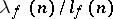can be estimated from below by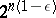,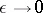as. Estimates of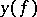— the so-called scatter of— have also been obtained. Here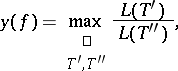whereand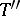are arbitrary dead-end disjunctive normal forms realizing, and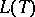is the number of characters in the dead-end disjunctive normal form. Examples of Boolean functions withhave been constructed; it has been established, however, that for "almost-all" Boolean functions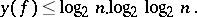The estimates above give a fairly complete idea of the difficulties that arise when Boolean functions are minimized according to the scheme: perfect disjunctive normal form — abridged disjunctive normal form — dead-end disjunctive normal form — minimal disjunctive normal form.